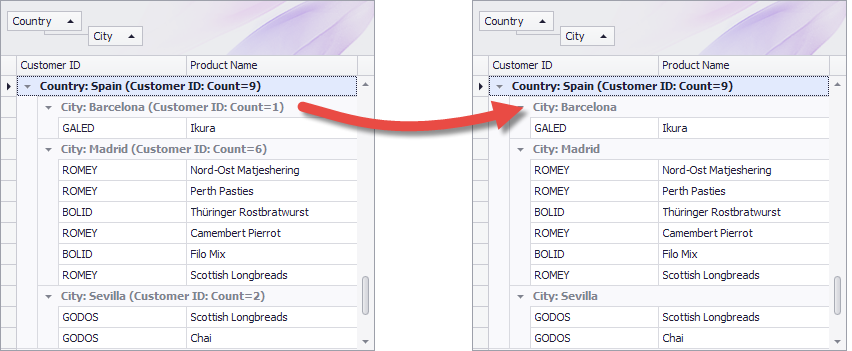20.2
20.1
19.2
19.1
18.2
18.1
17.2

# GridView.CustomSummaryExists Event

Enables you to specify which summaries should be calculated and displayed.

Namespace: DevExpress.XtraGrid.Views.Grid

Assembly: DevExpress.XtraGrid.v18.2.dll

## Declaration

``````[DXCategory("Data")]
public event CustomSummaryExistEventHandler CustomSummaryExists``````
``````<DXCategory("Data")>
Public Event CustomSummaryExists As CustomSummaryExistEventHandler``````

## Event Data

The CustomSummaryExists event's data class is CustomSummaryExistEventArgs. The following properties provide information specific to this event:

Property Description
Exists Gets or sets whether the summary value should be calculated and displayed.
GroupLevel Gets the nesting level of the group whose summary value is being calculated.
GroupRowHandle Gets a value identifying the group row whose summary value is about to be calculated.
IsGroupSummary Gets whether a group summary value is about to be calculated.
IsTotalSummary Gets whether a total summary value is about to be calculated.
Item Gets a summary item whose value is about to be calculated.

## Remarks

The event is raised before a particular summary value is calculated. There are two cases depending on the summary type:

The example below illustrates how to skip calculating summaries for a specific column.

``````
item1.SummaryType = DevExpress.Data.SummaryItemType.Count;
item1.Tag = 1;

//Add a group summary for the "Extended Price" column
item2.FieldName = "ExtendedPrice";
item2.SummaryType = DevExpress.Data.SummaryItemType.Sum;
item2.Tag = 2;
item2.DisplayFormat = "Sum={0:c2}";

// Do not calculate the Sum function (Tag=2) for City group rows
private void gridView1_CustomSummaryExists(object sender, DevExpress.Data.CustomSummaryExistEventArgs e) {
GridView view = sender as GridView;
int summaryTag = Convert.ToInt32((e.Item as GridSummaryItem).Tag);
GridColumn groupColumn = view.GroupedColumns[e.GroupLevel];
if (groupColumn.FieldName == "City" && summaryTag == 2) {
e.Exists = false;
}
}
``````

## Examples

By default, the Data Grid calculates group summaries (GridView.GroupSummary) for each group level. The following example handles the GridView.CustomSummaryExists event to allow a Count group summary to be only calculated for the root level and prevent it from being calculated for nested data groups.

The image below demonstrates the result.``````using DevExpress.XtraGrid;

private void gridView1_CustomSummaryExists(object sender, DevExpress.Data.CustomSummaryExistEventArgs e) {
GridGroupSummaryItem item = e.Item as GridGroupSummaryItem;
if (item == null) return;
if (e.GroupLevel > 0 && item.SummaryType == DevExpress.Data.SummaryItemType.Count)
e.Exists = false;
}
``````